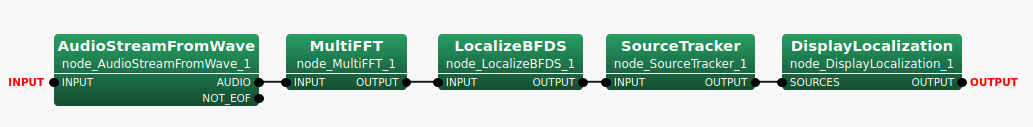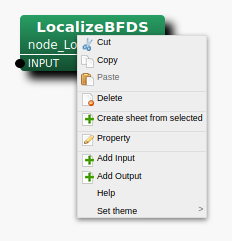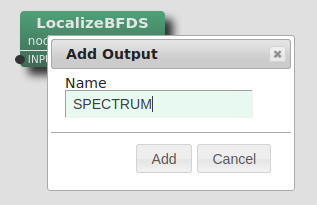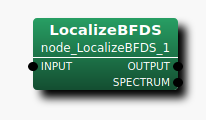## 6.2.22 LocalizeBFDS

### 6.2.22.1 Outline of the node

This estimates a sound’s direction using delay-and-sum (DS) beamforming (BF) method from multichannel waveform data.

### 6.2.22.2 Necessary file

The transfer function file or the position files is required. The transfer function file consists of a steering vector. The position files are the microphone position file and source position file which are created by harktool. When the position files are used, this node generates the transfer function based on the positional relationship between the microphone and sound.

### 6.2.22.3 Usage

When to use

This node estimates a sound’s direction using delay-and-sum beamforming (DS) method. This node is made for the test of EstimateTF node, so the output doesn’t have the enough information for post-processing such as tracking and source separation.

Typical connection

Figure 6.54 shows a typical connection example.Figure 6.54: Connection example of LocalizeBFDS

### 6.2.22.4 Input-output and property of the node

Input

INPUT

: Matrix<complex<float> > , Complex frequency representation of input signals with size $M \times (NFFT/2+1)$.

Output

OUTPUT

: Source position (direction) is expressed as Vector<ObjectRef>  type. ObjectRef  is a Source  and is a structure which consists of the BF power of the source and its direction. The element number of Vector  is a sound number ($N$), but $N=1$.

SPECTRUM

: Vector<float>  type. BF spectrum for every direction. This output terminal is not displayed by default.

Refer to Figure 6.55 for the addition method of hidden output.Step 1: Right-click LocalizeBFDS and click Add Output.Step 2: Enter SPECTRUM in the input, then, click Add.Step 3: The SPECTRUM output terminal is added to the node.
Figure 6.55: Usage example of hidden outputs : Display of SPECTRUM terminal

Parameter

Table 6.39: Parameter list of LocalizeBFDS
 Parameter name Type Default value Unit description TF_CHANNEL_SELECTION See below. Channel number used SAMPLING_RATE 16000 [Hz] Sampling rate LENGTH 512 [pt] FFT points ($NFFT$) USE_TF_FILE false Using flag for Transfer function file TF_FILENAME Transfer function file name MIC_POSITIONS Microphone position file name SRC_POSITIONS Source position file name SPEED_OF_SOUND 340 [m/s] Speed of sound DEBUG false ON/OFF of debug output
TF_CHANNEL_SELECTION

: Vector<int>  type. Of steering vectors of multichannel stored in the transfer function file, it is parameters which chooses the steering vector of specified channel to use. The channel number begins from 0 like ChannelSelector . Signal processing of 8 channel is assumed by default and it is set as <Vector<int> 0 1 2 3 4 5 6 7> . It is necessary to align the number ($M$) of elements of the parameters with the channel number of incoming signals. Moreover, it is necessary to align the order of channel and the channel order of TF_CHANNEL_SELECTION to be inputted into INPUT terminal.

SAMPLING_RATE

: int  type. 16000 is the default value. Sampling frequency of input acoustic signal. It is necessary to align with other nodes like LENGTH.

LENGTH

: int  type. 512 is the default value. FFT point in the case of fourier transform. It is necessary to align it with the FFT points to the preceding paragraph.

USE_TF_FILE

: bool type. In the case of true, this node uses the transfer function from the transefer function file. In the case of false, this node uses the transfer function based on the positional relationship between the microphone and sound.

TF_FILENAME

: string ． There is no default value. The file name of the transfer function file is designated. This parameter is shown when ${\rm USE\_ TF\_ FILE}=true$.

MIC_POSITIONS

: string . There is no default value. The file name of the microphone position file is designated. This parameter is shown when ${\rm USE\_ TF\_ FILE}=false$.

SRC_POSITIONS

: string . There is no default value. The file name of the source position file is designated. This parameter is shown when ${\rm USE\_ TF\_ FILE}=false$.

SPEED_OF_SOUND

: float  type. 340 is default value. The speed of sound.

DEBUG

: bool  type. ON/OFF of the debug output and the format of the debug output are as follows. First, the set of index of sound, direction, and power is outputted in tab delimited for only several number of sound detected in frames. Then, BF spectrum of the frame is shown.

### 6.2.22.5 Details of the node

Localization using delay-and-sum beamforming :

In the localization using delay-and-sum beamforming, the spatial spectrum is estimated by scanning the beam for the every direction. The beam is formed by following steps.

1. add the delay for the particular direction to each input signal.

2. sum the delayed signals.

In this node, the spatial spectrum $P(\theta )$ can be expressed as follows with the steering vector ${\boldsymbol w}^ H$ and the input signal ${\boldsymbol x}$.

 \begin{equation} \label{eq:LocalizaBFDS-eq1} P(\theta ) = {\boldsymbol w}^ H(\theta ) {\boldsymbol x} \end{equation} (32)

${\boldsymbol w}(\theta )$ for the direction $\theta$ can be expressed as follows with the transfer function ${\boldsymbol a}(\theta )$ .

 \begin{equation} \label{eq:LocalizaBFDS-eq2} {\boldsymbol w} (\theta ) = \frac{{\boldsymbol a}(\theta )}{|{\boldsymbol a}(\theta )|} \end{equation} (33)

Transfer function besed on the positional relationship between the microphone and sound :

In this node, the transfer function besed on the positional relationship between the microphone and sound is calculated assuming plane wave propagation.

### 6.2.22.6 References

• Futoshi Asano, “Array signal processing for acoustics” Acoustical Society of Japan